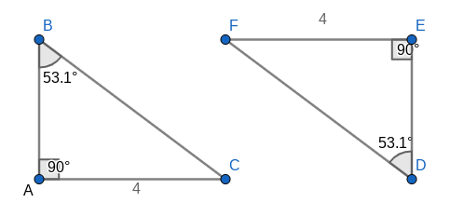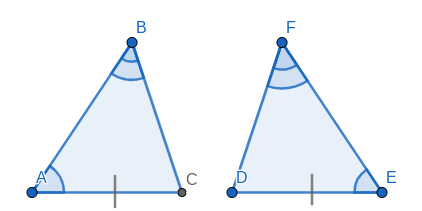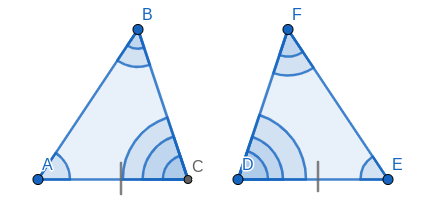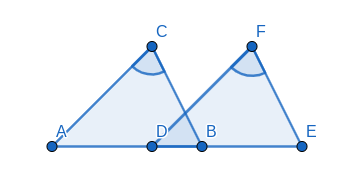# What is Angle-Angle-Side Congruence? Proof and Examples

Emma May, Jeff Calareso
• Author
Emma May

Emma May is a mathematician with a bachelor's degree in mathematics from Vassar College. She has tutored subjects such as calculus, linear algebra, and multivariable calculus for over three years. She also conducted mathematics research in topics such as combinatorics and dynamics for over four years.

• Instructor
Jeff Calareso

Jeff teaches high school English, math and other subjects. He has a master's degree in writing and literature.

Angle-angle-side (AAS) congruence is used to prove two triangles are congruent. The proof of AAS congruency is simple, and examples are included. Updated: 12/16/2021

Show

## AAS Theorem

The angle-angle-side congruency, or AAS, is a theorem that allows the determination of whether two triangles are congruent. Two triangles are congruent if they have three sides of the same length and three angles of the same measure. The theorem states that if two triangles have two equal angles and one side adjacent to only one of the angles, then the two triangles are congruent.The two triangles above share an equal side adjacent, and the two angles are equal. The equal side is only adjacent to one of the angles that are equal between the two triangles. This is why it is known as angle-angle-side: there are two angles next to each other and then one side next to only one of the angles. It reads as angle, angle, side. This is different from angle-side-angle, or ASA, where the sides that are equal are between the two angles. In order to use AAS, all that is necessary is identifying two equal angles in a triangle, then finding a third side adjacent to only one of the angles in each of the triangles such that the two sides are equal. This is enough to prove the two triangles are congruent.An error occurred trying to load this video.

Try refreshing the page, or contact customer support.

Coming up next: The HA (Hypotenuse Angle) Theorem: Proof, Explanation, & Examples

### You're on a roll. Keep up the good work!

Replay
Your next lesson will play in 10 seconds
• 0:05 Finding Matches
• 1:28 The AAS Theorem
• 3:12 Practice Proof
• 4:20 Finding Congruence
• 5:52 Lesson Summary
Save Save

Want to watch this again later?

Timeline
Autoplay
Autoplay
Speed Speed

## AAS Proof

The proof of the AAS congruence theorem is simple. To start, consider two triangles ABC and DEF such that there are two equal angles and one equal side adjacent to only one of the angles.As above, the angle A equals and angle E, and the angle B equals angle F. Notice that because the sum of the interior angles of a triangle equal 180 degrees, it is true that A + B + C = 180, so C = 180 - A - B = 180 - (A + B), and D + E + F = 180, so D = 180 - E - F = 180 - (E + F). Furthermore, A + B = E + F. Thus, it is true that angle C has the same measure as angle D. This means that there are two equal angles with an equal side between them.Therefore, by the ASA congruency theorem, the triangle ABC is congruent to the triangle DEF, because A = E, C = D, and |AC| = |DE|.

## AAS (Angle-Angle-Side) Property

There are two necessary properties for two triangles to be congruent by angle-angle-side. First, the two triangles must have two equal angles. Then, there must be a side adjacent to only one of the angles in each triangle such that the sides are equal. AAS is not used when the equal side is between the two angles. In that case, use the angle-side-angle, or ASA, theorem. If there are three equal sides, then use the side-side-side, or SSS, theorem. If there are two sides that are equal and one angle between the two sides that are equal, then use the side-angle-side, or SAS, theorem. It is important to note that if there are two equal sides and an angle adjacent to only one of those sides, known as side-side-angle or SSA, then the two triangles may not be congruent. Furthermore, if there are only two equal angles and no other information is given, it is impossible to determine whether the two triangles are congruent.

## Examples of AAS (Angle-Angle-Side)

Consider two triangles arranged like so:To unlock this lesson you must be a Study.com Member.

#### What is the AAS Theorem?

The theorem states that if two triangles have two equal angles and an equal side on each triangle adjacent to only one of the equal angles, then the two triangles are congruent.

#### What is the AAS congruency rule?

The AAS, or angle-angle-side, congruency rule states that if two triangles have two equal angles and a side adjacent to only one of the angles that are equal, then the two triangles are congruent.

#### What is the angle angle side rule?

The rule states that if two triangles share two equal angles and a side that is equal in each triangle, but is only adjacent to one of the two equal angles, then the triangles are congruent.

#### What is AAS give two example?

Consider two triangles ABC and DEF lied side by side such that AD = BE , and angle C equals angle F, and BC is parallel to EF. Then the two triangles are congruent.

Or, consider two triangles ABC and BDE arranged in a bowtie such that AB is parallel to DE and B is the midpoint of AE. Then the two triangles are congruent.

If two angles and a side are given, then it is possible to use the AAS rule if the two angles are equal and the length of the sides are equal.

### Register to view this lesson

Are you a student or a teacher?